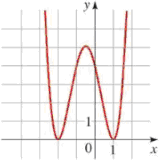Chapter 3.3, Problem 73EPrecalculus: Mathematics for Calcu...

7th Edition
James Stewart + 2 others
ISBN: 9781305071759

Solutions

Chapter
SectionPrecalculus: Mathematics for Calcu...

7th Edition
James Stewart + 2 others
ISBN: 9781305071759
Textbook Problem

Finding a Polynomial from a Graph Find the polynomial of the specified degree whose graph is shown.73. Degree 4To determine

The 4 degree polynomial from the given graph.

Explanation

Factor theorem states that the value of a given polynomial P(x) is zero at (x=c) if (xc) is a factor of P(x) . And its converse is also true, it states that (xc) is a factor of the polynomial P(x) , if and only if P(x)=0 at (x=c) .

The graphical representation of the square function, that is f(x)=[A(x)]2 , at point (x=0) is given as,

Figure 1

And the graphical representation of a polynomial is,

Figure 2

From the graph, the given polynomial P(x) is zero (or meets the xaxis ) at two different point as (2 and 1)

Still sussing out bartleby?

Check out a sample textbook solution.

See a sample solution

The Solution to Your Study Problems

Bartleby provides explanations to thousands of textbook problems written by our experts, many with advanced degrees!

Get Started

Sketch the graphs of the equations in Exercises 512. yx=1

Finite Mathematics and Applied Calculus (MindTap Course List)

Find the limit: limx3x2+x12x3

Calculus: An Applied Approach (MindTap Course List)

Find the median for the following set of scores: 1, 9, 3, 6, 4, 3, 11, 10

Essentials of Statistics for The Behavioral Sciences (MindTap Course List)

In Problems 1-20, evaluate the improper integrals that converge.

Mathematical Applications for the Management, Life, and Social Sciences

The graph of f(x) = 3x5 − 5x3 is:

Study Guide for Stewart's Single Variable Calculus: Early Transcendentals, 8th